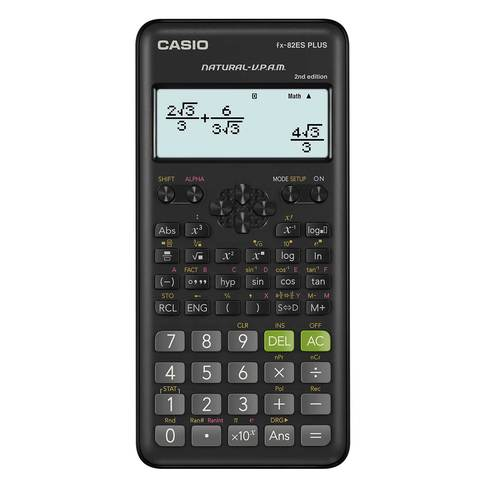HomeCasio

# Casio Scientific Calculator FX-82ES PLUS 2nd Edition

Sold by
Carrefour

## Technical details

Power
Power source
Battery type: 1 x AAA
Display
Digits
10
Display number of lines
2

## Description

The Natural Display (Natural-V.P.A.M.) shows mathematical expressions like roots and fractions as they appear in your textbook, and this increases comprehension because results are easier to understand.

Display type: 31 X 96 FULL DOT, Natural display, Algebraic input logic: Natural V.P.A.M. Number of characters/lines: 16/1+10/1. Significand function: 10+2

Memory- Repeat function, Variable memory: 9

Basic mathematics-Functions: 252, Bracket levels: 24, Fraction calculations, Angle dimensions DEG/RAD/GRAD. Conversions between >DEG/>RAD/>GRAD. Coordinate transformation Pol ÷ Rec. Trigonometric functions sin/cos/tan/sin-1/cos-1/tan-1. Hyperbolic functions sinh/cosh/tanh/sinh-1/cosh-1/tanh-1.Exponent, log, In, 10x, ex

Mathematical functions √, x2, x-1, 1/x, x!, xy, x1/y

Calculations in the sexagesimal system- Percentage calculations, Prime factorization, Technical notation ENG/ENG. Random number generator, Value table, Statistics, Summation of ∑x, ∑x2, Summation of ∑x, ∑y, ∑x2, ∑y2, ∑xy. Standard deviations for grouped and ungrouped values. Regression analysis, Permutations (nPr) and combinatorics (nCr). Differential and integral calculus. Other, Hardcase, Automatic shut-off.

Energy supply: Battery & type: 1x AAA

Size (H x W x D): 14mm x 77mm x 162mm

Weight: 105g